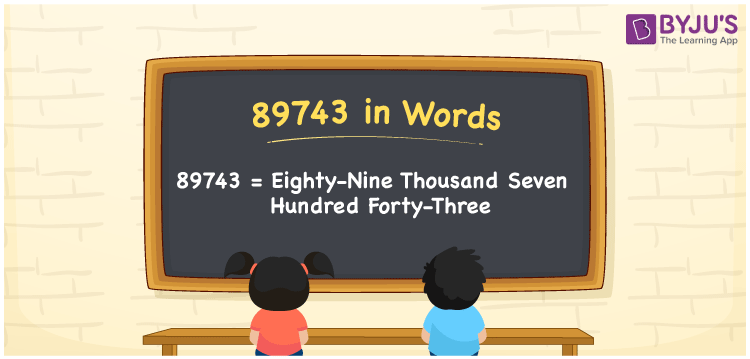# 89743 in Words

89743 in words is written as Eighty-nine thousand seven hundred forty-three. In both the International System of Numerals and the Indian System of Numerals, 89743 is written as Eighty-nine thousand seven hundred forty-three. The number 89743 is a Cardinal Number as it denotes some quantity. For example, “the boat costs 89743 rupees”.

 89743 in Words Eighty-nine thousand seven hundred forty-three Eighty-nine thousand seven hundred forty-three in Number 89743

## 89743 in English Words

89743 in English words is read as “Eighty-nine thousand seven hundred forty-three”.## How to Write 89743 in Words?

To write 89743 in words, we shall use the place value chart. In the place value chart, put 8 in the ten thousands, 9 in the thousands, 7 in the hundreds, 4 in the tens and 3 in the ones. Let us make a place value chart to write the number 89743 in words.

 Ten Thousands Thousands Hundreds Tens Ones 8 9 7 4 3

Thus, we can write the expanded form as

8 × Ten Thousand + 9 × Thousand + 7 × Hundred + 4 × Ten + 3 × One

= 8 × 10000 + 9 × 1000 + 7 × 100 + 4 × 10 + 3 × 1

= 80000 + 9000 + 700 + 40 + 3

= 89743

= Eighty-nine thousand seven hundred forty-three.

89743 is a natural number, the successor of 89742 and the predecessor of 89744.

89743 in words – Eighty-nine thousand seven hundred forty-three

• Is 89743 an odd number? – Yes
• Is 89743 an even number? – No
• Is 89743 a perfect square number? – No
• Is 89743 a perfect cube number? – No
• Is 89743 a prime number? – No
• Is 89743 a composite number? – Yes

## Frequently Asked Questions on 89743 in Words

Q1

### How to write 89743 in words?

89743 in words is written as Eighty-nine thousand seven hundred forty-three.
Q2

### How to write 89743 in the International and Indian System of Numerals?

In both, the system of numerals, 89743 in words, is written as Eighty-nine thousand seven hundred forty-three.
Q3

### How to write 89743 in a place value chart?

In the place value chart, write 8 in the ten thousands, 9 in the thousands, 7 in the hundreds, 4 in the tens and 3 in the ones, respectively.